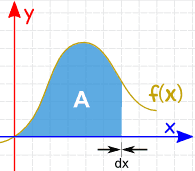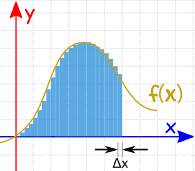﻿ Integral Approximation Calculator- Mathauditor

# Integral Approximation Calculator

Integration, as we all know, is a method to calculate areas by adding consecutive slices together. It is one of the best ways to find the area of any curve drawn in between the axis. You might be thinking that such a large Integration is highly impossible to do. Integration approximation calculator method lets you calculate the approximate answer by using easy integration techniques.##### Example

Let f(x)=ln(x) such that x varies from x=1 to x=4.The above integration is actually possible, and the actual solution to the above integration is 2.5451774.
We can also perform the above calculations by just calculating the value of log at every point. Such as-
at x=1 ln(x)= ln(1) =0
at x=2 ln(x)= ln(2) = 0.693147
And so on.
Note: You can even divide the whole graph into smaller slices with value even lesser than 1.